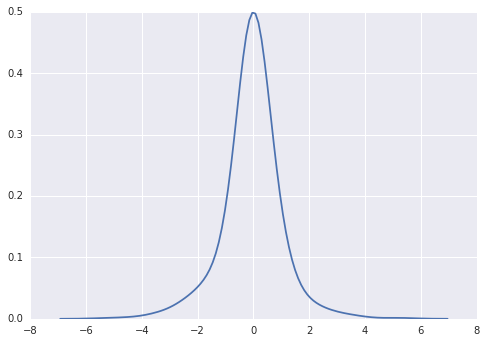#### 两个独立的正态随机变量的乘积服从什么分布？

统计/机器学习 概率分布    浏览次数：13229        分享

3MeganC   2017-03-22 11:49

##### 1个回答
4

$X\sim N(0,1)$，$Y\sim N(0,1)$并且$X$和$Y$独立。要确定它们的乘积$XY$的概率分布，我们可以通过正态分布的密度函数来计算出$XY$的密度函数。

\begin{eqnarray*}f_{XY}(z)&=&\int_{-\infty}^{\infty}\int_{-\infty}^{\infty}\frac{e^{-x^2/2}}{\sqrt{2\pi}}\frac{e^{-y^2/2}}{\sqrt{2\pi}}\delta(xy-z)dxdy\\&=&\frac{K_0(|z|)}{\pi}\end{eqnarray*}$$XY = \frac{(X+Y)^2 - X^2 - Y^2}{2}.$$

$X$和$Y$都是正态分布，所以$X+Y$是正态分布。所以可见$XY$其实是一个卡方分布减去两个卡方分布的结果。

SofaSofa数据科学社区DS面试题库 DS面经雷猴   2017-03-24 12:30相关主题